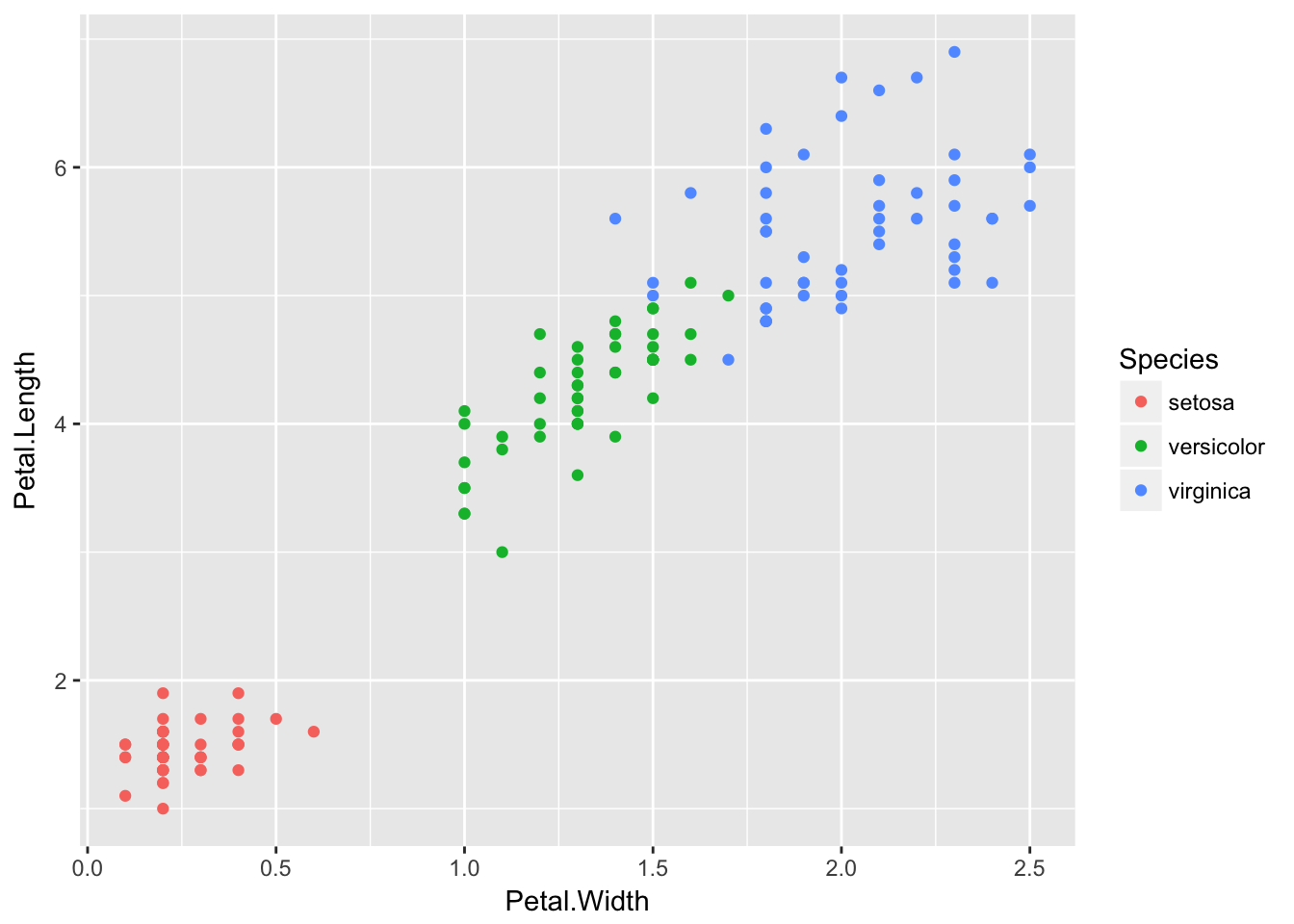# 1 Goal

The goal of this tutorial is to learn how to define the breaks in a geom point plot in ggplot.

# 2 Data import

``````# First we load the libraries
library(ggplot2)

# In this example we will use the open repository of plants classification Iris.
data("iris")
str(iris)``````
``````## 'data.frame':    150 obs. of  5 variables:
##  \$ Sepal.Length: num  5.1 4.9 4.7 4.6 5 5.4 4.6 5 4.4 4.9 ...
##  \$ Sepal.Width : num  3.5 3 3.2 3.1 3.6 3.9 3.4 3.4 2.9 3.1 ...
##  \$ Petal.Length: num  1.4 1.4 1.3 1.5 1.4 1.7 1.4 1.5 1.4 1.5 ...
##  \$ Petal.Width : num  0.2 0.2 0.2 0.2 0.2 0.4 0.3 0.2 0.2 0.1 ...
##  \$ Species     : Factor w/ 3 levels "setosa","versicolor",..: 1 1 1 1 1 1 1 1 1 1 ...``````
``````# Let's plot the petal length vs petal width
ggplot() + geom_point(data = iris, aes(x = Petal.Width, y = Petal.Length,color = Species))``````# 3 Define the breaks on both axis

``````# We can use scale x continuous to define the breaks in the x axis
# Let's define breaks every 0.25
ggplot() + geom_point(data = iris, aes(x = Petal.Width, y = Petal.Length,color = Species)) +
scale_x_continuous(breaks = seq(from = 0, to = 2.5, by = 0.25))``````